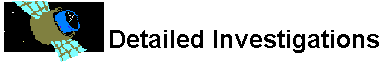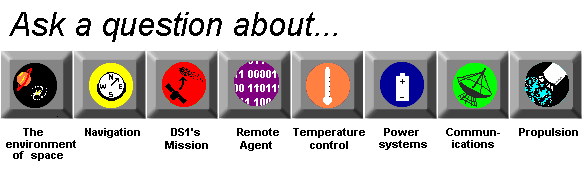# Propulsion

## What is a mole?

Moles are a unit of measurement for chemicals, just as meters are measurement units for length and grams are measurement units for mass. When a chemist or engineer wants to make a chemical reaction happen, he or she wants to make sure there is the right amount of each kind of chemical. Moles are used so that chemists, scientists or engineers know how much of different kinds of chemicals to use to have reactions that work out right.

A chemical can be atoms of a single element or atoms of many elements combined into molecules, so a single molecule of a chemical can weigh three or four times what a single molecule of another chemical weighs. For this reason, chemists can't just measure the weight of different chemicals to have the right proportions of reactants.

When chemists want to have a chemical reaction come out they need to know how many molecules of each kind of chemical they have, so they measure the chemicals in moles. A mole is the atomic weight of a molecule of the chemical in grams. So a mole of a molecule like hydrogen (H) with an atomic weight of 1 is one gram. Meanwhile, a complex molecule like glucose (C6H12O6) has an atomic weight of 180, so one mole is 180 grams. But even though the weight is different, the two moles contain the exact same number of molecules, 6.02 x 10 to the 23rd power.What is a propellant?How could something as small as an atom move a space craft?What is an atom?How do things burn?Why is mass important?What is mass?How do we measure what fuels weigh?What is specific impulse?